UP PREV NEXT

### 7. Mapping to library cells using an alternative area algorithm

Initial circuit Timing overview Best stage effort Library mapping Gate retiming Input buffering Better accuracy Prior art Summary Conclusions

#### Two coefficient area mapping

The patent refers to a single variable CS derived from the library data for each function and used to link the delay of a function to its area. As already seen, this leads to significant inaccuracies in the area estimate.

A better estimate of the area can be made by using two coefficients for the area approximation. This isn't described in the patent, but is an extension of the ideas given there. We replace the expression for the delay d:

d = τ × (p + COUT /(CS×A))
with
d = τ × (p + COUT /(CS0+CS1×A))

The two fitted curves for pin a of the 2-NAND gate are shown in the graph to the right. The curve labelled Est.Area2 uses two coefficients and is a better fit than curve Est.Area which uses a single coefficient.

The equation linking the estimated cell area to the delay d using two area coefficients is then:

A = {COUT /( d/τ -p)-CS0 }/CS1

Values for CS0 and CS1 are averaged over all the cells for a function. The constant timing model sets each cell's delay, and from this and the load capacitance COUT an estimate of its area according to the equation above. The area is mapped to a drive strength as shown in the example below for pin a of a 2-XOR gate.

Deriving the drive strength from the estimated cell area, pin a of a 2-XOR gate
CS0 = -3.14, CS1 = 0.67, g = 1.65
x05     x1     x2     x3     x4
CIN    3.34     5.34     10.30     15.07     20.29
Adjusted CIN    2.97     5.34     10.22     16.23     21.32
Actual width    8     8     15     19     25
Est. width A=CIN/(gCS1) - CS0 /CS1    7.4     9.6     14.0     19.5     24.1
Mapping  0   x05  8.4 x1 11.6 x2 16.5 x3 21.7 x4

The adjusted CIN compensates for differences in logical effort between the different cells. If as an example the estimated area is 8 tracks, which lies between 0 and 8.4, then the mapped cell is the xor2v0x05.

The graph below for an inverter shows that the fit between actual and estimated areas is much better than before, especially for the weaker drive strengths.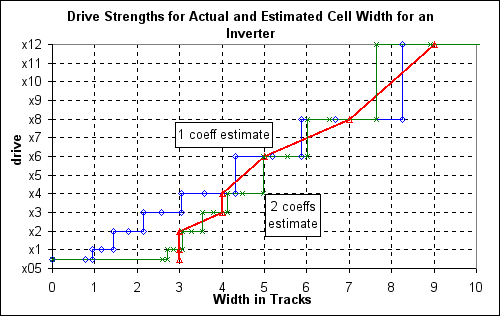The values of the single coefficient CS are listed in the table below. CS is the drive per unit area, so is higher for an inverter and lower for more complex gates.

Values of CS by function and pin
bf1    cgi2    iv1     nd2     nr2    xnr2   xor2       aoi21  oai21
a  2.05 0.39 2.87 1.37 1.08 0.41 0.47    a1  0.55 0.81
b    0.38   1.38 1.04 0.43 0.48  a2  0.55 0.81
c    0.39            b  0.57 0.85

The values of CS0 and CS1 are determined by a library analysis and are shown in the tables below. These are used to estimate the area of each cell in the 4-bit adder using the area equation above.

 Values of CS0 by function and pin bf1 cgi2 iv1 nd2 nr2 xnr2 xor2 aoi21 oai21 a -7.30 -1.51 -8.94 -5.52 -2.38 -2.94 -3.14 a1 -2.48 -2.84 b -1.49 -5.57 -2.30 -3.11 -3.18 a2 -2.49 -2.84 c -1.53 b -2.10 -2.98 Values of CS1 by function and pin bf1 cgi2 iv1 nd2 nr2 xnr2 xor2 aoi21 oai21 a 2.89 0.48 4.30 2.01 1.26 0.58 0.67 a1 0.89 0.98 b 0.48 2.03 1.22 0.61 0.67 a2 0.89 0.98 c 0.49 b 0.86 1.03

One can observe a problem with the methodology by comparing the coefficients for the aoi21 and the oai21 cells. Both should be similar, but the aoi21 is worse. This is because there are only three drive strengths for the aoi21 and two of these have the same width. The coefficient values are quite sensitive to the number of drive strengths.

#### Netlist comparison

The drive strengths produced by mapping with one area coefficient and by two are the same for the adder with unbuffered inputs. For this circuit, the more accurate mapping has no benefit.

For the adder with buffered inputs there is an improvement in the final schematic. Fig 7a on the right shows the schematic with drive strengths mapped using 1 area coefficient and the critical path with vsclib timing of 371ps, 6% more than the spec. Fig 7b shows the schematic with drive strengths mapped using 2 area coefficients and idealised timing so that all critical paths are 350ps. Instance b0n has an x2 drive strength instead of x1 (Fig 6b). Fig 7c shows the vsclib timing using the drive strengths from Fig 7b. The critical path is 354ps, 1.1% above the spec.

The area estimates produced by either of the coefficients for this circuit is good, as shown in the table below.

Est.
CS
Actual
CS
Est.
CS0 CS1
Actual
CS0 CS1
adder gates   74.4 75.7 73.7 75.7
Fig 5c   Fig 5c
estimation error     1.7%   2.6%
buffered adder gates   87.5 86.7 86.8 86.7
Fig 6b Fig 7a Fig 7b Fig 7c
estimation error     1.0%   0.1%

The area estimate can give values which are larger than the largest cell or smaller than the smallest cell. In this case, the actual largest or smallest area is used instead.

Fitting single and double area coefficients for pin a of a 2-NAND gate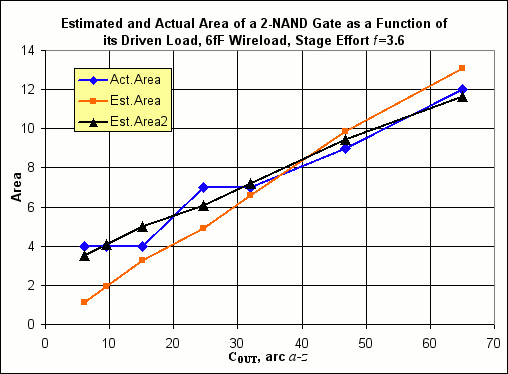Fig 7a. Buffered adder delays with (i) fixed stage effort of f=3.6 used for initial timing; (ii) each gate delay compressed or stretched to meet critical path; (iii) wireload of 6fF per fanout; (iv) gain limit of 5 used for non-inverting gates; (v) single area coefficient CS to map drive strength; (vi) timing from vsclib cells.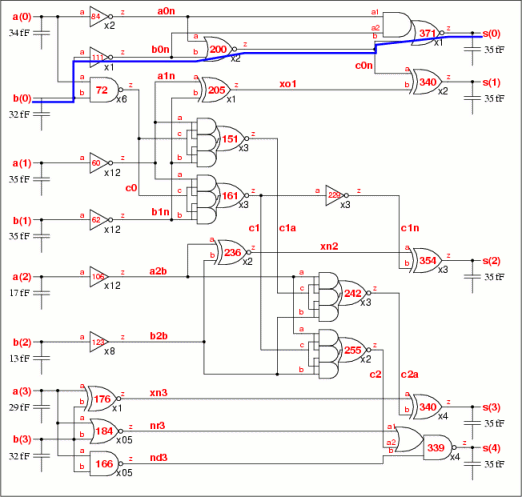Fig 7b. Buffered adder delays with (i) fixed stage effort of f=3.6 used for initial timing; (ii) each gate delay compressed or stretched to meet critical path; (iii) wireload of 6fF per fanout; (iv) two area coefficients CS to map drive strength; (v) timing using library averages.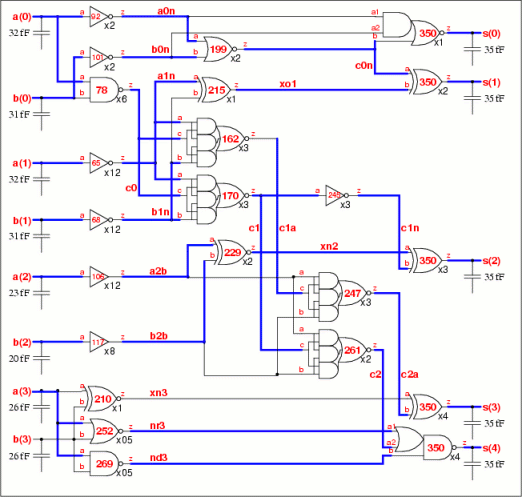Fig 7c. Buffered adder delays with (i) fixed stage effort of f=3.6 used for initial timing; (ii) each gate delay compressed or stretched to meet critical path; (iii) wireload of 6fF per fanout; (iv) gain limit of 5 used for non-inverting gates; (v) two area coefficients CS0,CS1 to map drive strength; (vi) timing from vsclib cells.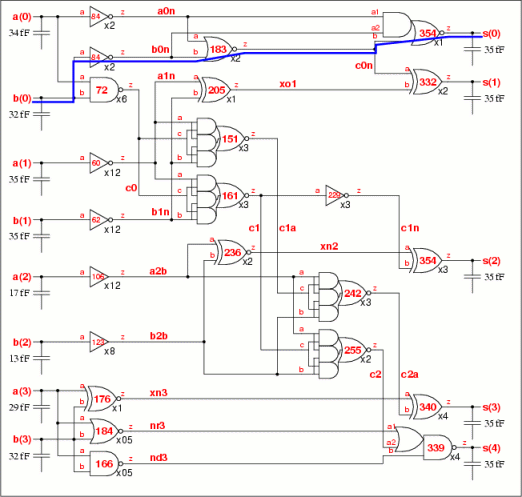UP PREV NEXT 5-AUG-05